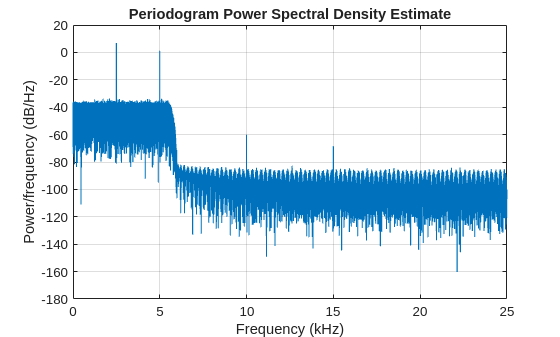Documentation

This is machine translation

Mouseover text to see original. Click the button below to return to the English version of the page.

fftfilt

FFT-based FIR filtering using overlap-add method

Syntax

```y = fftfilt(b,x) y = fftfilt(b,x,n) y = fftfilt(d,x) y = fftfilt(d,x,n) y = fftfilt(gpuArrayb,gpuArrayX,n) ```

Description

`fftfilt` filters data using the efficient FFT-based method of overlap-add, a frequency domain filtering technique that works only for FIR filters.

`y = fftfilt(b,x)` filters the data in vector `x` with the filter described by coefficient vector `b`. It returns the data vector `y`. The operation performed by `fftfilt` is described in the time domain by the difference equation:

`$y\left(n\right)=b\left(1\right)x\left(n\right)+b\left(2\right)x\left(n-1\right)+\cdots +b\left(nb+1\right)x\left(n-nb\right)$`

An equivalent representation is the Z-transform or frequency domain description:

`$Y\left(z\right)=\left(b\left(1\right)+b\left(2\right){z}^{-1}+\cdots +b\left(nb+1\right){z}^{-nb}\right)X\left(z\right)$`

By default, `fftfilt` chooses an FFT length and a data block length that guarantee efficient execution time.

If `x` is a matrix, `fftfilt` filters its columns. If `b` is a matrix, `fftfilt` applies the filter in each column of `b` to the signal vector `x`. If `b` and `x` are both matrices with the same number of columns, the ith column of `b` is used to filter the ith column of `x`.

`y = fftfilt(b,x,n)` uses `n` to determine the length of the FFT. See Algorithms for information.

`y = fftfilt(d,x)` filters the data in vector `x` with a `digitalFilter` object, `d`. Use `designfilt` to generate `d` based on frequency-response specifications.

`y = fftfilt(d,x,n)` uses `n` to determine the length of the FFT.

`y = fftfilt(gpuArrayb,gpuArrayX,n)` filters the data in the `gpuArray` object, `gpuArrayX`, with the FIR filter coefficients in the `gpuArray`, `gpuArrayb`. See Run MATLAB Functions on a GPU (Parallel Computing Toolbox) for details on `gpuArray` objects. Using `fftfilt` with `gpuArray` objects requires Parallel Computing Toolbox™ software. Refer to GPU Support by Release (Parallel Computing Toolbox) to see what GPUs are supported. The filtered data, `y`, is a `gpuArray` object. See Overlap-Add Filtering on the GPU for example of overlap-add filtering on the GPU.

`fftfilt` works for both real and complex inputs.

Comparison to `filter` function

When the input signal is relatively large, it is advantageous to use `fftfilt` instead of `filter`, which performs N multiplications for each sample in `x`, where N is the filter length. `fftfilt` performs 2 FFT operations — the FFT of the signal block of length L plus the inverse FT of the product of the FFTs — at the cost of

½Llog2L

where L is the block length. It then performs L point-wise multiplications for a total cost of

L + Llog2L = L(1 + log2L)

multiplications. The cost ratio is therefore

L(1+log2L) / (NL) = (1 + log2L)/N

which is approximately log2L / N.

Therefore, `fftfilt` becomes advantageous when log2Lis less than N.

Examples

collapse all

Verify that `filter` is more efficient for smaller operands and `fftfilt` is more efficient for large operands. Filter ${10}^{6}$ random numbers with two random filters: a short one, with 20 taps, and a long one, with 2000. Use `tic` and `toc` to measure the execution times. Repeat the experiment 100 times to improve the statistics.

```rng default N = 100; shrt = 20; long = 2000; tfs = 0; tls = 0; tfl = 0; tll = 0; for kj = 1:N x = rand(1,1e6); bshrt = rand(1,shrt); tic sfs = fftfilt(bshrt,x); tfs = tfs+toc/N; tic sls = filter(bshrt,1,x); tls = tls+toc/N; blong = rand(1,long); tic sfl = fftfilt(blong,x); tfl = tfl+toc/N; tic sll = filter(blong,1,x); tll = tll+toc/N; end```

Compare the average times.

`fprintf('%4d-tap filter averages: fftfilt: %f s; filter: %f s\n',shrt,tfs,tls)`
``` 20-tap filter averages: fftfilt: 0.289484 s; filter: 0.024282 s ```
`fprintf('%4d-tap filter averages: fftfilt: %f s; filter: %f s\n',long,tfl,tll)`
```2000-tap filter averages: fftfilt: 0.096268 s; filter: 0.354657 s ```

This example requires Parallel Computing Toolbox™ software. Refer to GPU Support by Release (Parallel Computing Toolbox) to see what GPUs are supported.

Create a signal consisting of a sum of sine waves in white Gaussian additive noise. The sine wave frequencies are 2.5, 5, 10, and 15 kHz. The sampling frequency is 50 kHz.

```Fs = 50e3; t = 0:1/Fs:10-(1/Fs); x = cos(2*pi*2500*t)+0.5*sin(2*pi*5000*t)+0.25*cos(2*pi*10000*t)+ ... 0.125*sin(2*pi*15000*t)+randn(size(t));```

Design a lowpass FIR equiripple filter using `designfilt`.

```d = designfilt('lowpassfir','SampleRate',Fs, ... 'PassbandFrequency',5500,'StopbandFrequency',6000, ... 'PassbandRipple',0.5,'StopbandAttenuation',50); B = d.Coefficients;```

Filter the data on the GPU using the overlap-add method. Put the data on the GPU using `gpuArray`. Return the output to the MATLAB® workspace using `gather` and plot the power spectral density estimate of the filtered data.

```y = fftfilt(gpuArray(B),gpuArray(x)); periodogram(gather(y),rectwin(length(y)),length(y),50e3)```Algorithms

`fftfilt` uses `fft` to implement the overlap-add method , a technique that combines successive frequency domain filtered blocks of an input sequence. `fftfilt` breaks an input sequence `x` into length `L` data blocks, where L must be greater than the filter length N.and convolves each block with the filter `b` by

```y = ifft(fft(x(i:i+L-1),nfft).*fft(b,nfft)); ```

where `nfft` is the FFT length. `fftfilt` overlaps successive output sections by `n-1` points, where `n` is the length of the filter, and sums them.`fftfilt` chooses the key parameters `L` and `nfft` in different ways, depending on whether you supply an FFT length `n` and on the lengths of the filter and signal. If you do not specify a value for `n` (which determines FFT length), `fftfilt` chooses these key parameters automatically:

• If `length(x)`is greater than `length(b)`, `fftfilt` chooses values that minimize the number of blocks times the number of flops per FFT.

• If `length(b)` is greater than or equal to `length(x)`, `fftfilt` uses a single FFT of length

```2^nextpow2(length(b) + length(x) - 1) ```

This essentially computes

```y = ifft(fft(B,nfft).*fft(X,nfft)) ```

If you supply a value for `n`, `fftfilt` chooses an FFT length, `nfft`, of `2^nextpow2(n)`and a data block length of `nfft` - `length(b)` + `1`. If `n` is less than `length(b)`, `fftfilt` sets `n` to `length(b)`.

References

 Oppenheim, Alan V., Ronald W. Schafer, and John R. Buck. Discrete-Time Signal Processing. 2nd Ed. Upper Saddle River, NJ: Prentice Hall, 1999.